# Basic

Basic electrical components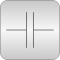# Package Contents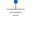Ground Ground node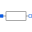Resistor Ideal linear electrical resistor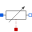HeatingResistor Temperature dependent electrical resistor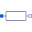Conductor Ideal linear electrical conductor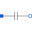Capacitor Ideal linear electrical capacitor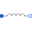Inductor Ideal linear electrical inductor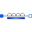SaturatingInductor Simple model of an inductor with saturation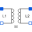Transformer Transformer with two ports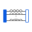M_Transformer Generic transformer with free number of inductors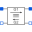Gyrator Gyrator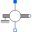EMF Electromotoric force (electric/mechanic transformer)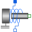TranslationalEMF Electromotoric force (electric/mechanic transformer)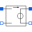VCV Linear voltage-controlled voltage source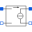VCC Linear voltage-controlled current source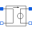CCV Linear current-controlled voltage source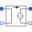CCC Linear current-controlled current source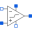OpAmp Simple nonideal model of an OpAmp with limitation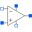OpAmpDetailed Detailed model of an operational amplifier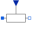VariableResistor Ideal linear electrical resistor with variable resistanceVariableConductor Ideal linear electrical conductor with variable conductance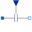VariableCapacitor Ideal linear electrical capacitor with variable capacitance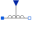VariableInductor Ideal linear electrical inductor with variable inductance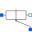Potentiometer Adjustable resistor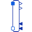GeneralCurrentToVoltageAdaptor Signal adaptor for an Electrical OnePort with voltage and derivative of voltage as outputs and current and derivative of current as inputs (especially useful for FMUs)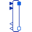GeneralVoltageToCurrentAdaptor Signal adaptor for an Electrical OnePort with current and derivative of current as output and voltage and derivative of voltage as input (especially useful for FMUs)

# Information

This information is part of the Modelica Standard Library maintained by the Modelica Association.

This package contains very basic analog electrical components such as resistor, conductor, capacitor, inductor, and the ground (which is needed in each electrical circuit description. Furthermore, controlled sources, coupling components, and some improved (but nevertheless basic) are in this package.